Ex 5.4 (Optional)

Chapter 5 Class 10 Arithmetic Progressions
Serial order wise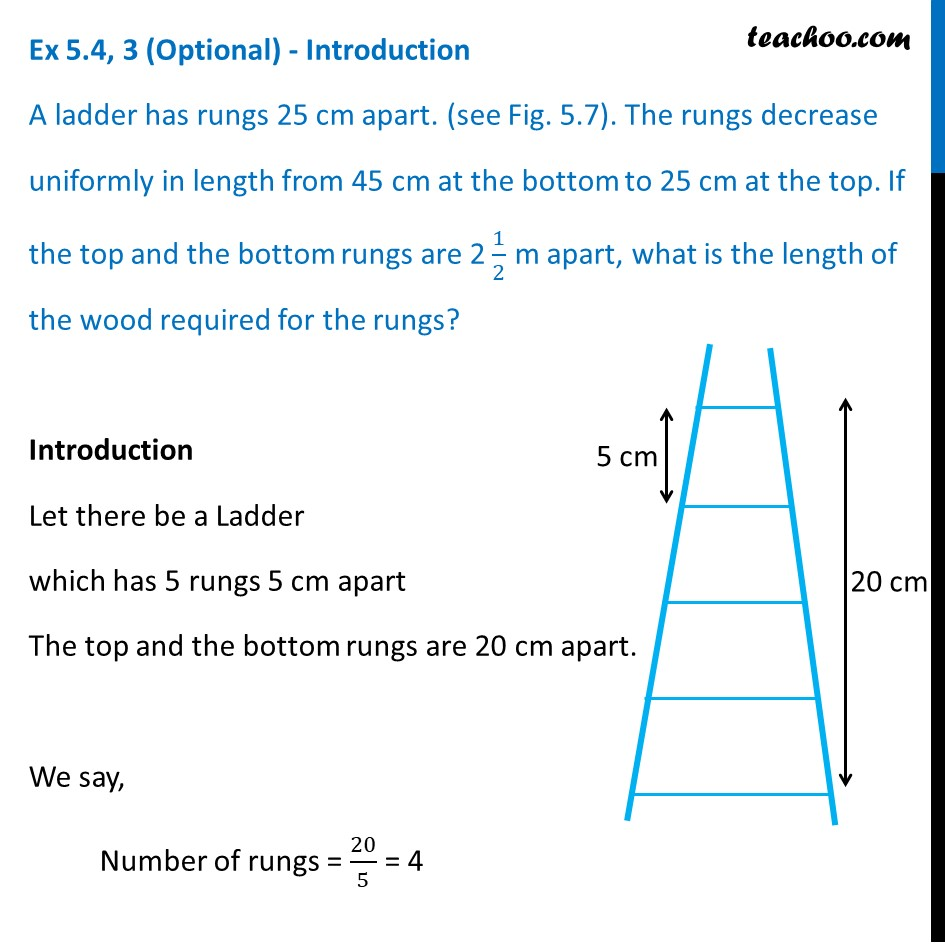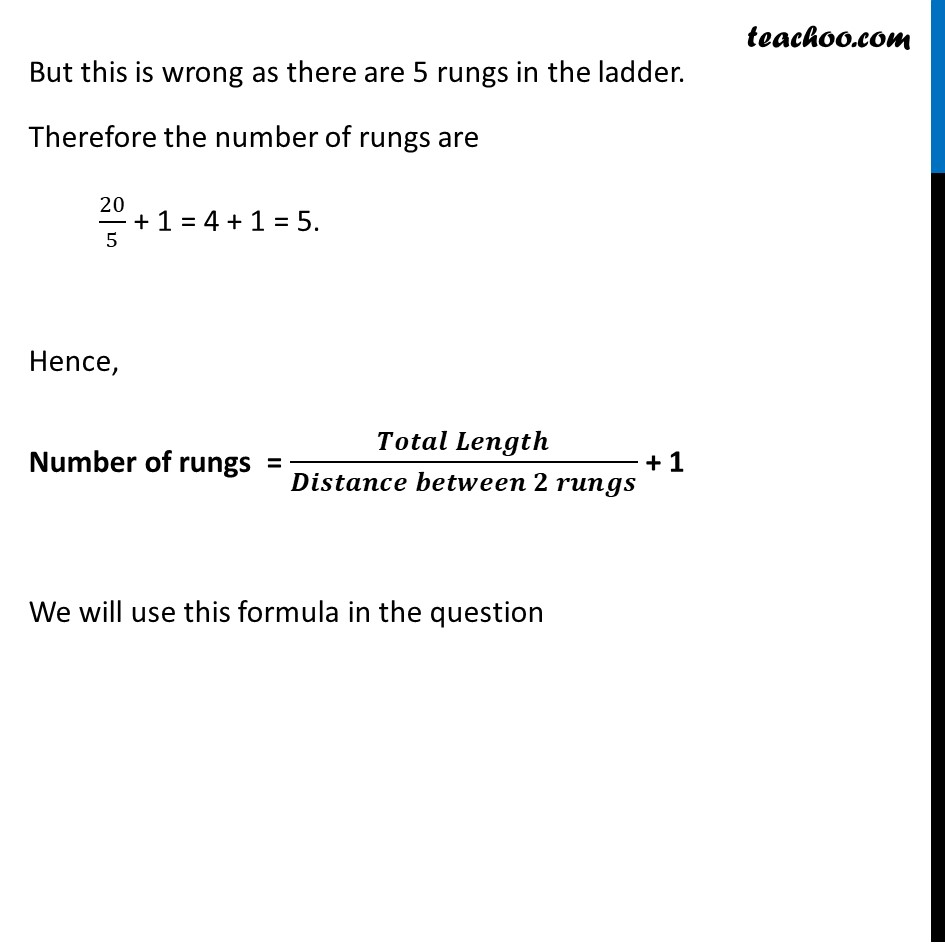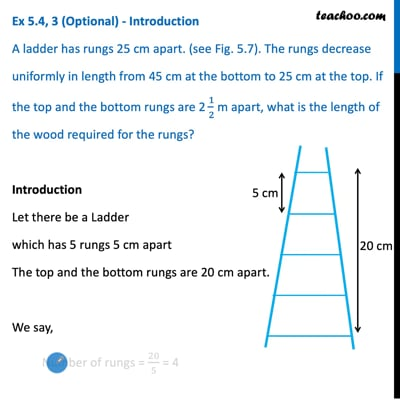This video is only available for Teachoo black users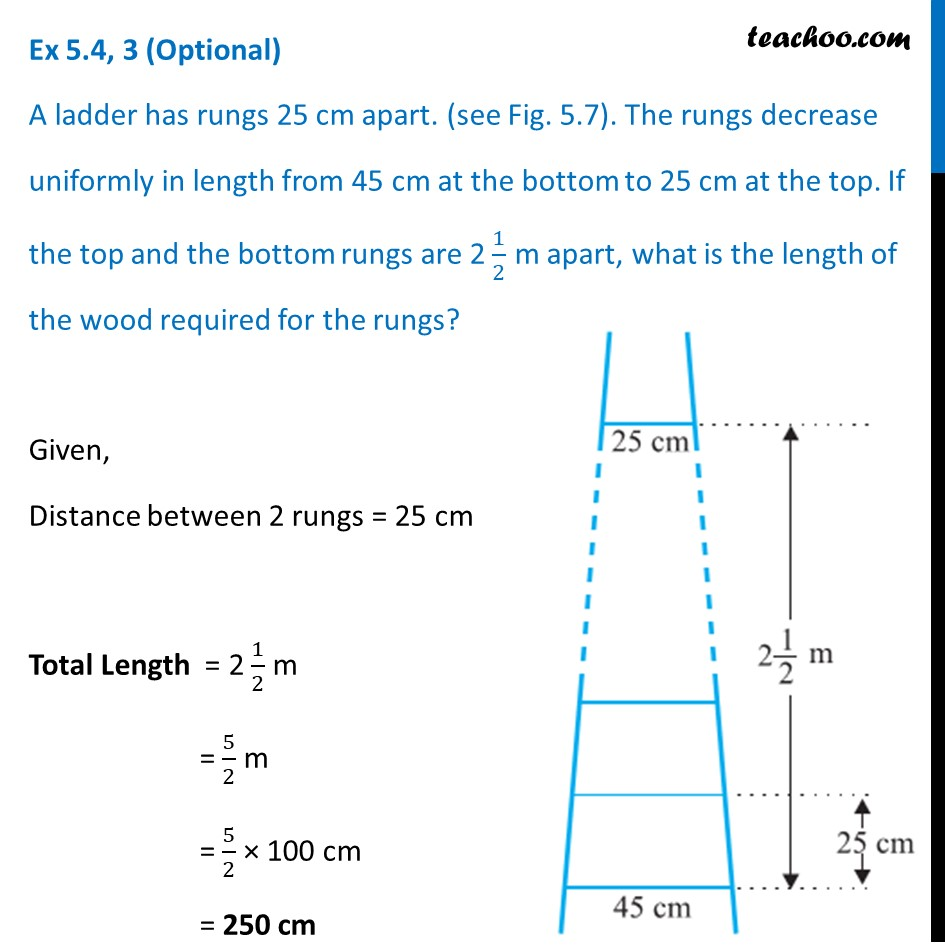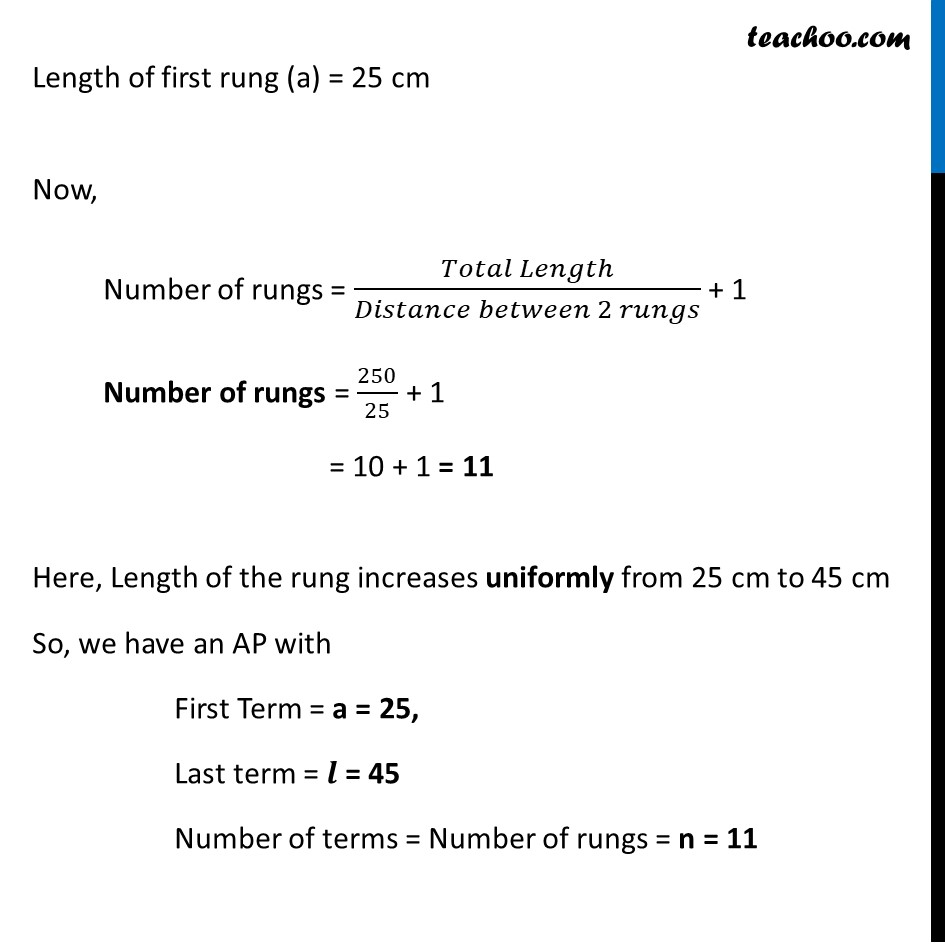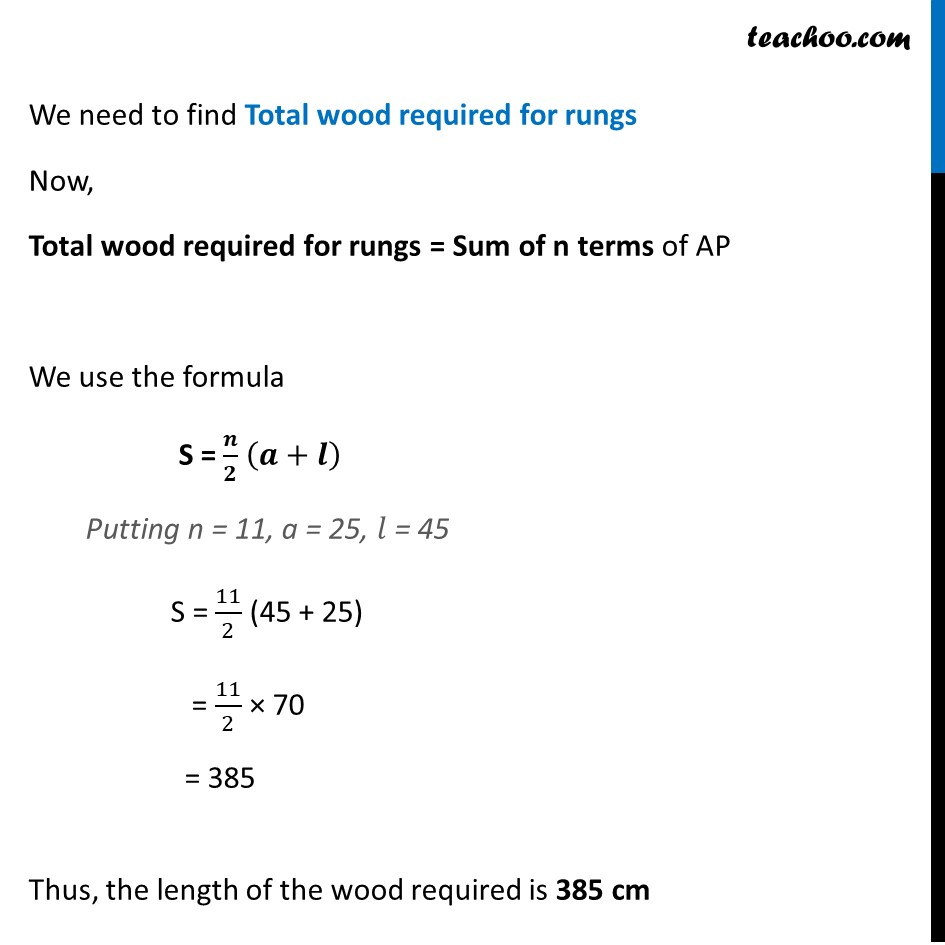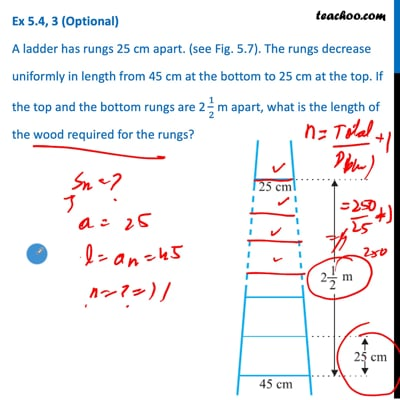This video is only available for Teachoo black users

Maths Crash Course - Live lectures + all videos + Real time Doubt solving!

### Transcript

Ex 5.4, 3 (Optional) - Introduction A ladder has rungs 25 cm apart. (see Fig. 5.7). The rungs decrease uniformly in length from 45 cm at the bottom to 25 cm at the top. If the top and the bottom rungs are 2 1/2 m apart, what is the length of the wood required for the rungs? Introduction Let there be a Ladder which has 5 rungs 5 cm apart The top and the bottom rungs are 20 cm apart. We say, Number of rungs = 20/5 = 4 But this is wrong as there are 5 rungs in the ladder. Therefore the number of rungs are 20/5 + 1 = 4 + 1 = 5. Hence, Number of rungs = (𝑻𝒐𝒕𝒂𝒍 𝑳𝒆𝒏𝒈𝒕𝒉)/(𝑫𝒊𝒔𝒕𝒂𝒏𝒄𝒆 𝒃𝒆𝒕𝒘𝒆𝒆𝒏 𝟐 𝒓𝒖𝒏𝒈𝒔) + 1 We will use this formula in the question Ex 5.4, 3 (Optional) A ladder has rungs 25 cm apart. (see Fig. 5.7). The rungs decrease uniformly in length from 45 cm at the bottom to 25 cm at the top. If the top and the bottom rungs are 2 1/2 m apart, what is the length of the wood required for the rungs? Given, Distance between 2 rungs = 25 cm Total Length = 2 1/2 m = 5/2 m = 5/2 × 100 cm = 250 cm Length of first rung (a) = 25 cm Now, Number of rungs = (𝑇𝑜𝑡𝑎𝑙 𝐿𝑒𝑛𝑔𝑡ℎ)/(𝐷𝑖𝑠𝑡𝑎𝑛𝑐𝑒 𝑏𝑒𝑡𝑤𝑒𝑒𝑛 2 𝑟𝑢𝑛𝑔𝑠) + 1 Number of rungs = 250/25 + 1 = 10 + 1 = 11 Here, Length of the rung increases uniformly from 25 cm to 45 cm So, we have an AP with First Term = a = 25, Last term = 𝒍 = 45 Number of terms = Number of rungs = n = 11 We need to find Total wood required for rungs Now, Total wood required for rungs = Sum of n terms of AP We use the formula S = 𝒏/𝟐 (𝒂+𝒍) Putting n = 11, a = 25, 𝑙 = 45 S = 11/2 (45 + 25) = 11/2 × 70 = 385 Thus, the length of the wood required is 385 cm